# Sentence Correction using Recurrent Neural Networks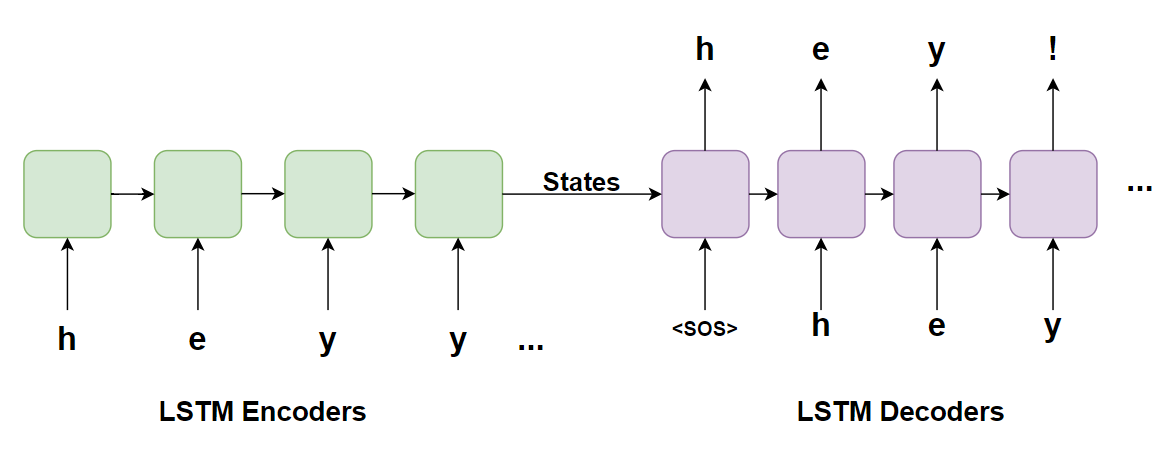Character level Sequence to Sequence Model

# Contents

2. DL Problem Formulation
4. Overview of Dataset
5. Performance Metric
6. Success Metric
7. Existing Solutions
8. My First Cut Approach
9. Exploratory Data Analysis
10. Data Preprocessing
11. Data Preparation for Model
12. Data Augmentation and Data Generation
13. Deep Learning Models
14. Summary
15. Deployment
16. Future Work
17. Code Repository
18. References

This Case Study on “Sentence Correction using Recurrent Neural Networks” is a research paper-based case study dealing with mapping text which have social media type of shortened texts to a proper English language text. The paper mainly deals with preprocessing of the raw text obtained from a public domain and converting it to texts which are close to English language. For example: “Lea u there?” should be converted to “Lea, are you there?”.

# Deep Learning Problem Formulation

The main aim of the case study is to correct or map ‘short typed’ English sentences without punctuations and grammatical sense to proper English based sentences with punctuations and grammar. This solution acts as a pre-processing method which can be used prior to a larger NLP model.

1. Provide clean English sentences from raw user input as part of pre-processing to feed to a larger NLP Model. Clean sentences will make a Model learn the rules of the language and hence provide better suggestions to a user.

2. There is no time limit for the corrections, but if the corrections are real time it can be used in prediction that can be suggested to the user while real time typing.

# Overview of Dataset

Data Source: The dataset is obtained from National University of Singapore (NUS) website http://www.comp.nus.edu.sg/~nlp/sw/sm_norm_mt.tar.gz. The dataset was created by the NLP group at NUS by randomly selecting 2000 SMS messages and normalizing them to formal English language and further translated to Chinese. Following Image is a sample of the first two Messages in the dataset.

The dataset contains three sentences with the first being the English sentence that may or may not need correction, the target English sentence with proper punctuation and grammar and the third sentence being the Chinese translated target sentence. Since we are dealing with the only English based translations, we will remove the Chinese translated sentences and use the first sentence as the input and the second sentence as the target.

# Performance Metric

As mentioned in the paper, we are using cross entropy loss function since the final vector is a 94D ASCII character vector with a SoftMax function deciding the output character and replicating the result.

The Metric evaluation during the model training phase would be ‘accuracy’ since the output is one hot encoded 94 Dimension vector of the visible ASCII characters and would be useful in determining whether the model is predicting the next character as expected from the target sentence.

# Success Metric

The success metric for the sentence correction would be predicting sentences that conform to English language and its rules. The metrics that can do the same are BLEU score, Absolute accuracy.

Absolute Accuracy = (total sentences that are perfectly corrected) / (total number of sentences in the test case)

Absolute Accuracy would help us determine if our model is actually correcting most of the sentences exactly as required since the main goal is to correct sentences.

# Existing Solutions

There are several solutions but only word level where as this solution deals at character level. There is a famous paper by Andrew Ng where there is a use of character level approach with Attention mechanism. The only disadvantage with the current research paper dataset is the size of dataset at mere 2000 where as the dataset by Andrew Ng had over 50,000 which is significant for training a model.

# My First Cut Approach

With the analysis of various blogs, research papers and codes mentioned in the previous sections, a broader picture in solving the problem was obtained. The dataset provided to us from the research paper is a small 2000 sentence dataset. My first cut approach would be to initially start implementing the research paper itself. The length of the input and target sentences can be analyzed. Remove sentences that are outliers and also check the BLEU score of the sentences of the input-output pairs.

The dataset can be augmented from the methods learnt in other blogs by taking the target sentences are generating random perturbations in the sentences and be able to obtain a dataset around 10k or more. It would also be good to add own known SMS language words to the target sentence and generate more sentences. The research paper is restricted to 1 layer and 2-layer model and adding more layers can increase the learning dynamics. Attention mechanism also can be added in order to understand its effect on the Character level as well as word level. For the metric, we can use BLEU score as a metric since the research paper deals with only cross entropy loss. Optimizers such as Adam can be used over that of mini batch SGD that has been already used in the research paper. The main model to start off with would be Encoder and Decoders using LSTM along with and without attention and vary the parameters and note the output scores. I would thus like to start with the above approach and then move towards other models and better ideas during the course of solving the problem.

# Exploratory Data Analysis

The dataset being small, there are only few visual data analysis that can be performed. The dataset containing sentences in casual and formal english language is checked for its character count in each sentence. The plot below is the character counts of input and target sentences and we see that both input and target sentences are almost of similar lengths.

`fig, axes = plt.subplots(1, 1, figsize=(7,7))sns.distplot(input_lengths, hist=False, ax=axes, label='Input Sentences')sns.distplot(target_lengths, hist=False, ax=axes, label='Target Sentences')axes.set_title("Distribution of lengths of sentences")fig.tight_layout()`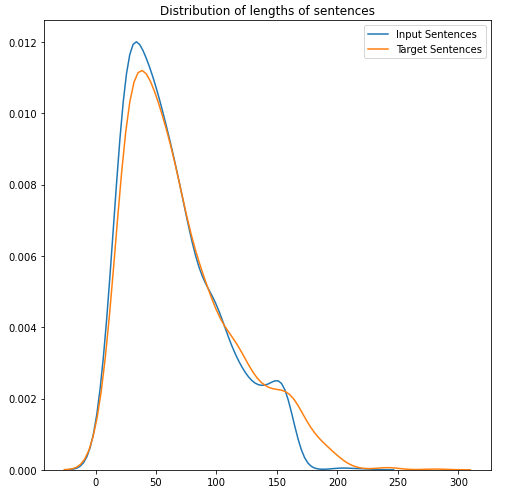Distribution of lengths of Input and Target sentences

The difference between casual language and Formal english language is that we tend not to use punctuations in casual SMS languages and this can be seen by keeping only special characters in sentence and then counting the lengths of the sentences.

`input_special_chars = []target_special_chars = []for i, row in data_df.iterrows():    input_sp_char = re.sub('[\w]+' ,'', row['input'])    target_sp_char = re.sub('[\w]+' ,'', row['target'])    input_special_chars.append(len(input_sp_char))    target_special_chars.append(len(target_sp_char))`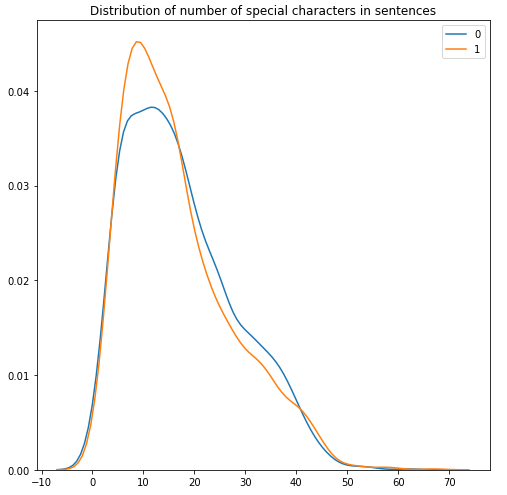Distribution of lengths of Special Characters in the Input and Target sentences

We see that the target sentences are usually have higher punctuation count since in SMS language we write in short forms and do not use punctuations. The lengthier sentences have similar special character count due to the excess use of “…” and “!!!” in casual SMS languages.

# Data Preprocessing

As part of Data preprocessing, we keep only sentences that have printable characters and also remove very long sentences that are comparatively outlier by restricting the length of input sentence below 170 and target sentences below 200.

`df_filtered = data_df[data_df['input_lengths'] < 170]df_filtered = df_filtered[df_filtered['target_lengths'] < 200]df_filtered = df_filtered[df_filtered['input_printable'] == True]df_filtered = df_filtered[df_filtered['target_printable'] == True]`

Due to this restriction we see that only 11 sentences are dropped from a set of 2000 sentences and hence we are left with 1989 sentences to work with.

# Data Preparation for Model

Before we prepare the data for the model, we need to split the data into train test split so as to not see the data before hand while preparations. Since we have a low dataset of 1989 we use a split of 99:1 which provides a train set of 1969 and test set of 20.

`X_train, X_test, y_train, y_test = train_test_split(X, y, test_size=0.01, shuffle=False)`

Since we are dealing with character level model, we need to set a start of sentence and end of sentences as characters. For this case study we choose “\t” for Start of Sentence and “\n” for End of Sentence.

`train['target_ip'] = '\t' + train['target'].astype(str)train['target_op'] =  train['target'].astype(str) + '\n'test['target_ip'] = '\t' + test['target'].astype(str)test['target_op'] =  test['target'].astype(str) + '\n'`

We then convert the data using a Tokenizer at character level and do not restrict with any filters as we have only a few characters of 94

`tokenizer_raw_ip = Tokenizer(    char_level=True,    lower=False,    filters=None)`

We then further fit the tokenizer on the train set so as to learn the unique characters in the input and target sentences.

`tokenizer_raw_ip.fit_on_texts(train['input'].values)tokenizer_target_ip.fit_on_texts(train['target_ip'].values)`

Using the trained tokenizers, we convert the characters into their respective numeric values using the tokenizers learned character dictionary. This is followed by padding the sequences to the length of the longest sequence of the input and also the target sentence separately.

`self.encoder_seq = self.tokenizer_raw_ip.texts_to_sequences([self.encoder_inps[i]])self.encoder_seq = pad_sequences(self.encoder_seq, maxlen=self.max_length_encoder, dtype='int32', padding='post')`

With this the data is ready to fed to the model as all the sentences are padded to the same lengths and characters are converted to their respective numeric values.

# Data Augmentation and Data Generation

Since there was limited dataset, a dictionary of over 1500 words were formed by manually mapping formal English words with Casual English words. For example: {“You”:[“U”, “u”, “Yu”, “uuu”, “YOU”,..]}

The target sentences were taken one at a time and since each formal word hand multiple options to select from, we chose the casual words randomly for the list of words from the dictionary.

So the following were the generated sentences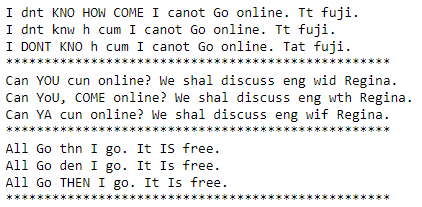Generating Sentences using Random Selection of casual words

The dataset was increased from 2000 to approximately 7000 and some words do not have any alternate casual words and hence the duplicate sentences from the dataset was removed.

Apart from this, various British Novel Text ‘Pride and Prejudice’, ‘war and peace’, ‘Shakespeare’ were extracted and cleaned. The cleaned sentences from the novel were chosen and replaced with the casual words for the input sentences and the sentences from the novel acted as target sentences. This way we obtained addition 2000 sentences and after data augmentation it provided over 4000 sentences. Different combinates of datasets were generated where few dataset had only the SMS dataset with augmentation, few with Novel and SMS dataset which generated around 3.7 Million sentences.

# Deep Learning Models

## 1. Encoder Decoder Model with 1 layer LSTM

The first model is a simple Encoder Decoder model where the Encoder consists of one embedding layer and one LSTM layer. The Embedding Layer is of size 20 since we have only 96 characters and LSTM units is set at 100 as per the research paper. Finally the output is passed through a Dense layer with a SoftMax activation function. This model was trained using Adam optimizer and Sparse Categorical Cross Entropy.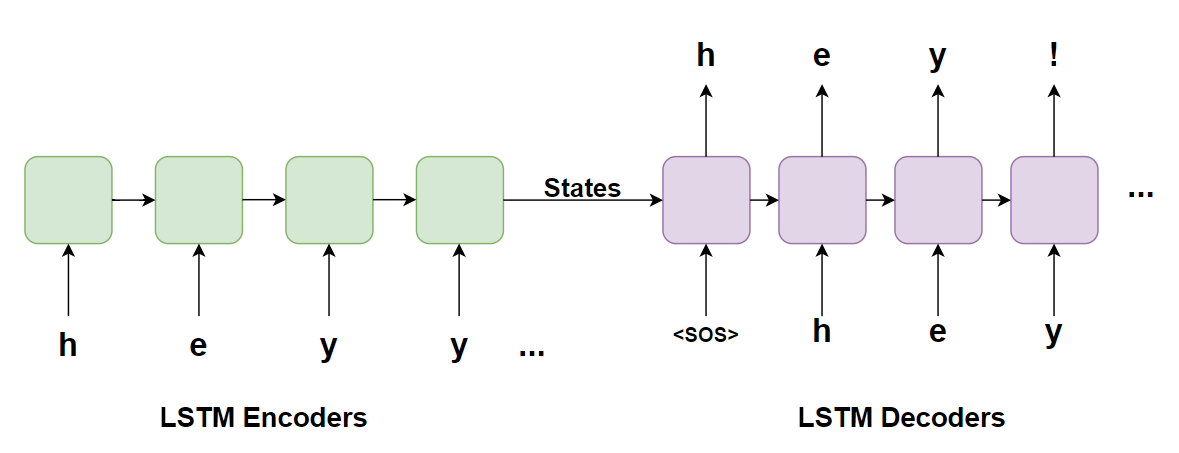1 Layer LSTM Encoder Decoder Model
`# Encoderinput_embedded = self.embedding(input_sentences)self.lstm_output, self.lstm_state_h,self.lstm_state_c = self.lstm(input_embedded)# Decodertarget_embedded        = self.embedding(target_sentences)lstm_output, _,_        = self.lstm(target_embedded, initial_state=[state_h, state_c])`

This model was able to provide a validation loss of 0.35 and thus a perplexity value of 1.27. The model performed well for smaller sentences and for longer sentences the output beyond initial few letters are random or not of significant meaning.

## 2. Encoder Decoder Model with 2 Layer LSTM

As per the research paper, we have implemented 2 layer LSTM Encoder Decoder Model where the Encoder States of Layer 2 is passed on to the LSTMs of Decoder Layer 1 and Layer 2 as shown in the below figure.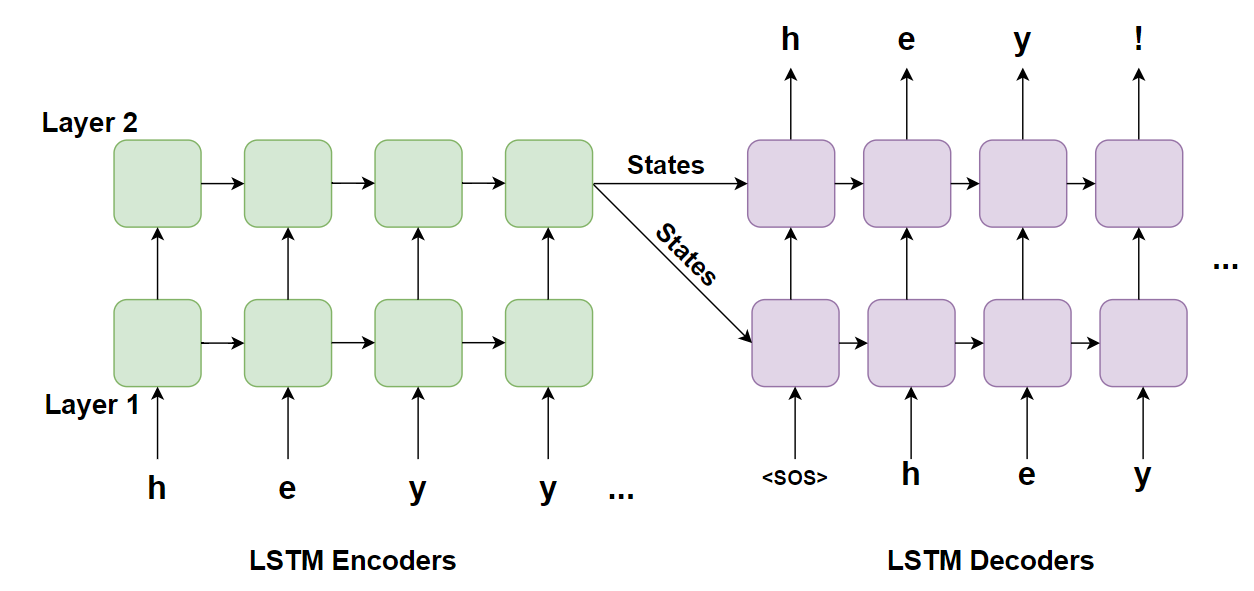2 Layer LSTM Encoder Decoder Model
`# Encoderinput_embedded = self.embedding(input_sentences)self.lstm_output_1, lstm_state_h_1,self.lstm_state_c_1 = self.lstm_1(input_embedded)self.lstm_output_2, self.lstm_state_h_2,self.lstm_state_c_2 = self.lstm_2(self.lstm_output_1# Decodertarget_embedd = self.embedding(target_sentences)lstm_output_1, decoder_state_h_1, decoder_state_c_1 = self.lstm_1(target_embedd, initial_state=[state_h, state_c])lstm_output, _ ,_        = self.lstm_2(lstm_output_1, initial_state=[state_h, state_c])`

The Optimizer used for this model was Adam with a Learning rate of 0.01 and using ReduceLROnPlatue with a factor of 0.8 and patience of 3. The loss used was the Sparse Categorical Cross Entropy. The number of LSTM units in each of the Layers and Encoder/Decoder was 100. But several other combinations were tried such as varying embedding size from 20 to 100, LSTM Units from 50 to 400 were tried and the best results were obtained for 100 LSTM Units and Embedding Size of 20. The perplexity value for this model was at 1.25 which is similar to the previous model and the output sentences did not have significant improvement other than a few corrections in punctuations

.

## 3. Attention Model with Luong General Global Attention

Inspired by the Andrew Ng Paper using the Character Level Attention mechanism, the same was tried for this model. Luong’s General Attention was used as part of the Global Attention for the Encoder Outputs. The Attention layer uses a Feed Forward Neural Network to learn the weightage of each of the letters in the Encoder Outputs and uses it as the Context Vector along with input of the Decoder. The states of the Encoder are passed to the Decoder as usual as the previous models.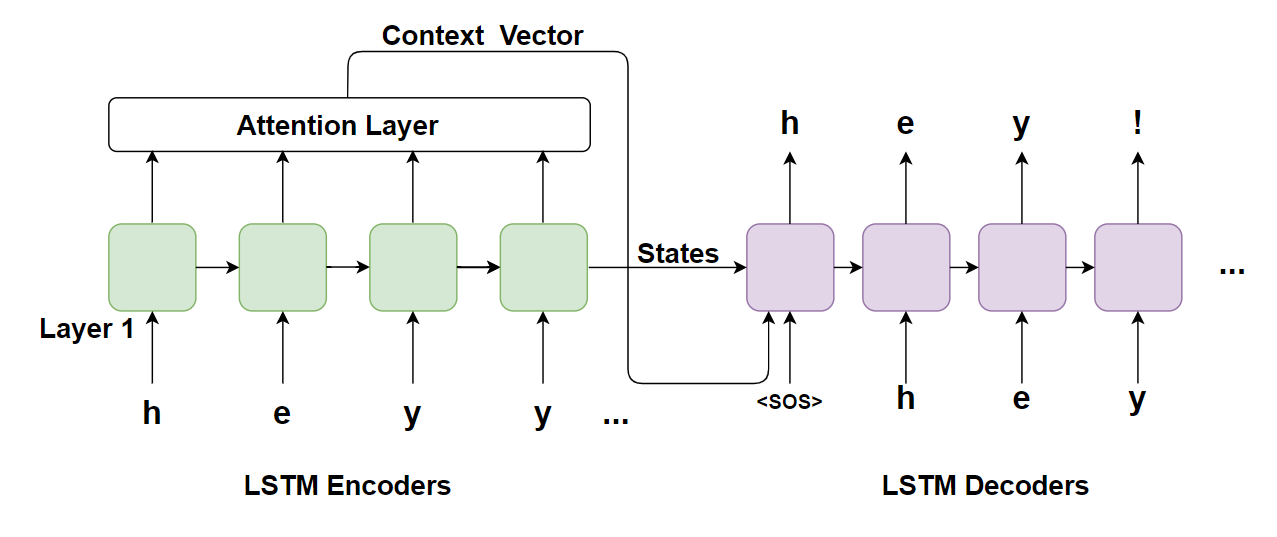Attention Model with 1 Layer LSTM Encoder and Decoder Model
`# Encoderembedding = self.encoder_embedding_layer(input_sequence)self.encoder_output, self.hidden_state, self.cell_state = self.encoder_lstm_layer(embedding)# Decoderfor timestep in range(DECODER_OUTPUT_LEN):output, decoder_h, decoder_c, attention_weights, context_vector = self.one_step_decoder(input_to_decoder[:,timestep:timestep+1], encoder_output, decoder_hidden_state, decoder_cell_state)`

The model was trained for 100 epochs with Adam optimizer at Learning Rate of 0.01 and reduced using callback when there was no improvement in Validation loss for more than 3 epochs. The Embedding size was 20 and the number of LSTM units were 100. The perplexity value was significantly higher compared to the previous model due to the lack of larger data and stood at 1.65. The output was not great even for shorter sentences due to larger perplexity value.

## 4. Encoder Decoder Model with One Hot Encoded Vectors

The final model that was tried was by removing the Embedding Layer and using One Hot Encoded vectors. One hot coded vectors are converted from numerical data to a dimension of the number of characters. For example, if there are totally 5 characters then each of them can be represented as 10000, 01000, 00100, 00010, 00001, where each character is represented by a unique vector where only one of the value is 1 and rest zero. This helps us in changing the loss function from Sparse Categorical Cross Entropy to just Categorical Cross Entropy.

Categorical Cross Entropy is much more useful since the probability value is provided for all the outputs for a given vector and thus helping is learning better through backpropagations.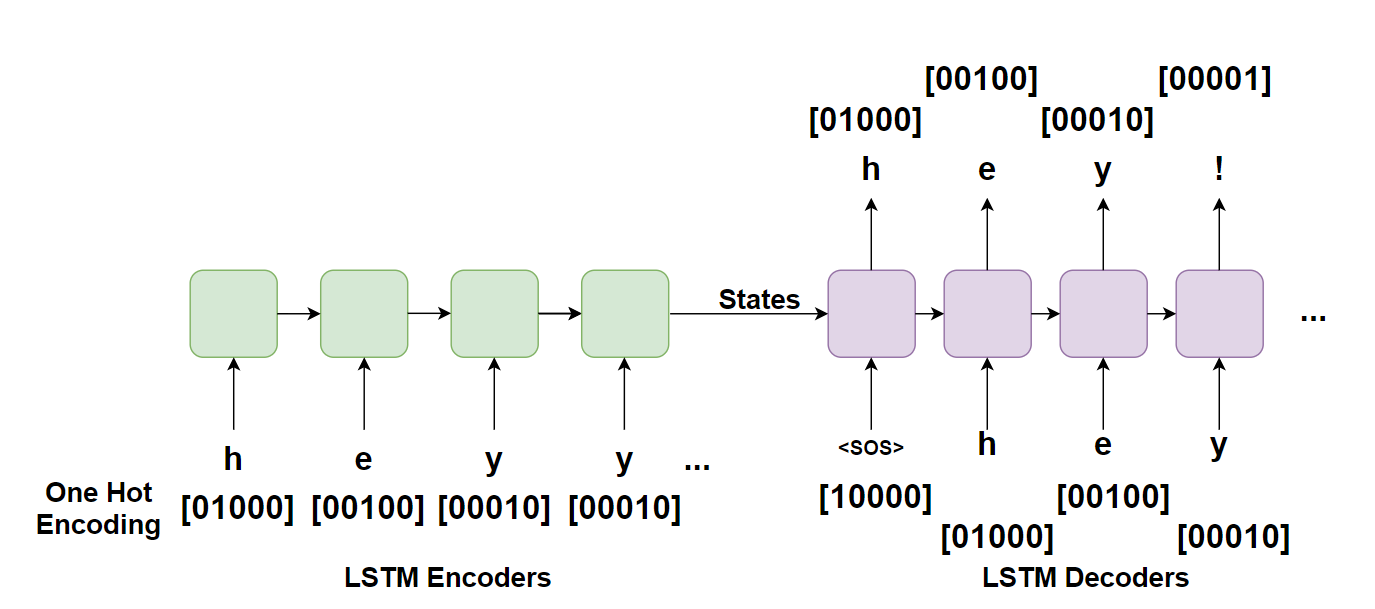1 Layer LSTM Encoder Decoder Models with One Hot Encoded Vector Inputs
`# Encoder self.lstm_output, self.lstm_state_h,self.lstm_state_c = self.lstm(input_sentances)# Decoderlstm_output, _,_        = self.lstm(target_sentences, initial_state=[state_h, state_c])`

The model here was trained with Adam optimizer at Learning rate of 0.01 and Categorical Cross Entropy. The model performed slightly better than all other models with final perplexity value at 1.23. The outputs though are similar since there is only a marginal improvement. The number of epochs here was just 20 and beyond that the model starts overfitting significantly and the validation loss keeps increasing. In this Model even 2 Layer LSTM Encoder Decoder Models with One Hot Encoding was tried but results were quite similar with perplexity at 1.28.

## 5. Encoder Decoder Model with 2 LSTM layer in Encoder, 1 LSTM layer in Decoder

This unique model was used for mainly sentence correction of non-SMS dataset used till now. Several novels were taken and split for short sentences with maximum length of 70 characters. These sentences were introduced with 4 types of errors mainly random letter deletion, letter addition, letter exchange and letter substitution.

With the above dataframe, unigrams were extracted from both input and target sentences and a 1:1 dataframe was made which contains error words as input and corrected words as target. The Encoder Decoder Model mentioned in the heading was trained on this model with words rather than sentences. The words training performed much better due to the small lengths and Encoder Decoder Models known to perform well for shorter sentences. During the error introduction, we introduced only 3 errors in sentences before creating the word dataframe. During prediction, we take input as a sentence, break into words and pass each word and obtain character level prediction for the word. Once a single word is predicted we move on the next word and thus we are able to predict whole sentence character wise. The model was able to correct 2 errors for most of the sentences and all 3 for very few.

## 6. Other Model Combinations

Several other models were tried and test for the dataset and the results were almost similar or worse than the above 4 models.

a. 3 Layer LSTM Encoder Decoder Model

b. 4 Layer LSTM Encoder Decoder Model

c. 3 Layer LSTM Encoder Decoder Model with One Hot Encoding Input

d. 4 Layer LSTM Encoder Decoder Model with One Hot Encoding Input

The outputs of higher layered models were not good as the output was random and no meaning could be found. This is mainly due to smaller dataset and the Model was not able to learn anything much from them. These higher Layer models become to complex for a simple dataset of 2000 sentences.

All the models above were tried with 6 different datasets namely,

a. Dataset of SMS
b. SMS + Augmented SMS Data
c. SMS + Augmented Novel Data
d. Pure Novel Dataset
e. Pure Novel Dataset + Augmentation with SMS language
f. Pure Novel Dataset + Augmentation with typo mistakes, letter addition/deletion, letter exchanges

The best results obtained for the Last dataset with the Novel sentences and the error introduction in the sentences where we generated up to 3.7 Million sentences using data augmentation. The least perplexity value obtained was at 1.06 for the One Hot Encoded Vectors with 2 LSTM layer in Encoder and 1 LSTM layer in Decoder.

# Summary

Overall we see that the results for shorter sentences with characters less than ~20 are very good and the models are able to convert and correct the words from SMS language to formal English. The 1 layer model overall performed better than the higher layers due to the smaller dataset and less complexity of the dataset.

The Attention models did not perform very well as expected due to the limitation of the dataset. The one hot encoded vector model performed similar to the output of character encoded vector model and the perplexity ranged from 1.23 to 1.27. The data augmentation from target sentences and ‘Pride and Prejudice’ Novel was able to reduce the loss marginally but nothing significant. The Model with 2 LSTM layers in Encoder and 1 LSTM layer in Decoder trained on the 3.7M sentences generated by error introduction in the Novel dataset performed the best with least perplexity of 1.06.

All the results expect the last one is for dataset a,c,e mentioned in the previous section. The last result is for the dataset f which had the least loss and perplexity value.

# Deployment

The solution was deployed using Streamlit locally on the system and the code is available on GitHub.

The input should be sentence of shorter length so as to get good results. For longer sentences since the model has not learnt much the output is expected to be with lack of meaning.Output of Sentence correction using RNN

# Future Work

The Case study was done using LSTM RNNs and can be tried with GRU. Since the dataset is small, if there is an availability of training character models we can try using Transfer Learning to make it perform better.

Currently there are no datasets available apart from the 2000 sentences and thus it would be good to generate new data without augmentation and use it to improve the model.

--

--